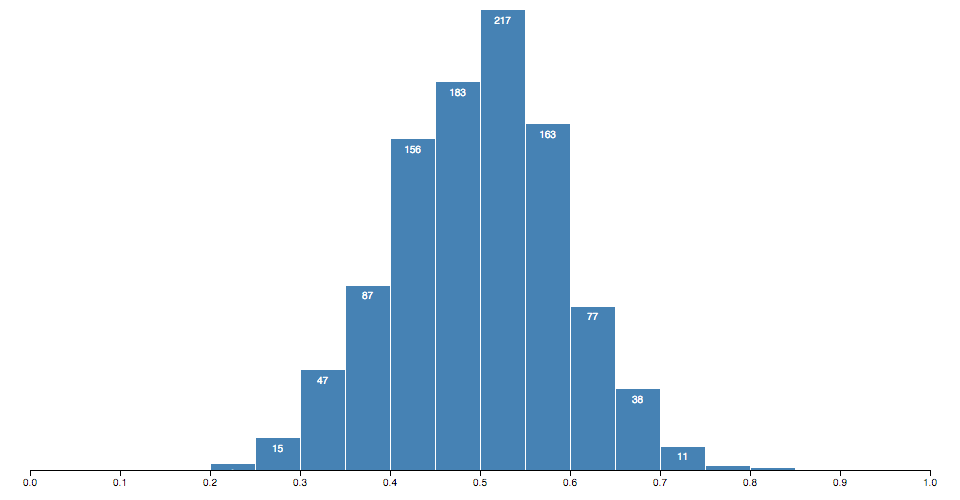d3-array, array 操作，排序，搜索，汇总等

Array manipulation, ordering, searching, summarizing, etc.

源代码名称:d3-array源代码网址:http://www.github.com/d3/d3-arrayd3-array源代码文档d3-array源代码下载Git URL: ``git://www.github.com/d3/d3-array.git``Git Clone代码到本地: ``git clone http://www.github.com/d3/d3-array``Subversion代码到本地: ``````\$ svn co --depth empty http://www.github.com/d3/d3-array Checked out revision 1. \$ cd repo \$ svn up trunk ``````

d3-array

JavaScript中的数据通常由 array 表示，因这里可以在可以视化或者分析数据时操作数组。 一些常见的操作形式包括采用一个连续的切片( 子集)，使用谓词函数过滤一个，并使用转换函数将一个 array 映射到一个并行的值集合。 先查看这个模块提供的实用程序集，然后熟悉内置的强大的 array 方法以实现 JavaScript。

JavaScript包含了的变异方法，用于修改 array:

finally 对 array 中元素应用函数的迭代方法:

• 使用predicate创建一个新的array，只包含一个谓词，其中包含一个谓词。
• array foreach - 调用 array 中的每个元素。
• 每个元素都有一个值，每个元素都满足一个谓词。
• 使用调用函数的结果创建一个新的array，该结果在 array 中的每个元素调用一个函数。
• 确定是否至少有一个元素满足谓词的条件。
• 将函数缩减为单个值( 来自 left-to-right )的方法。
• 将函数应用到单个值( 来自 right-to-left ) 中。

安装

```<scriptsrc="https://d3js.org/d3-array.v1.min.js"></script>
<script>var min =d3.min(array);</script>```

API参考

统计

# d3.min(array [, accessor] ) <>

# d3.max(array [, accessor] ) <>

# d3.extent(array [, accessor] ) <>

# d3.sum(array [, accessor] ) <>

# d3.mean(array [, accessor] ) <>

# d3.median(array [, accessor] ) <>

# d3.quantile(array, p [, accessor] ) <>

`var a = [0, 10, 30];d3.quantile(a, 0); // 0d3.quantile(a, 0.5); // 10d3.quantile(a, 1); // 30d3.quantile(a, 0.25); // 5d3.quantile(a, 0.75); // 20d3.quantile(a, 0.1); // 2`

# d3.variance(array [, accessor] ) <>

# d3.deviation(array [, accessor] ) <>

全文搜索

# d3.scan(array [, comparator] ) <>

`var array = [{foo:42}, {foo:91}];d3.scan(array, function(a, b) { returna.foo-b.foo; }); // 0d3.scan(array, function(a, b) { returnb.foo-a.foo; }); // 1`

# d3.bisectLeft(array, x [, lo[, hi] ]) <>

# d3.bisect(array, x [, lo[, hi] ]) <>
# d3.bisectRight(array, x [, lo[, hi] ]) <>

# d3.bisector(accessor) <>
# d3.bisector(comparator) <>

```var data = [
{date:newDate(2011, 1, 1), value:0.5},
{date:newDate(2011, 2, 1), value:0.6},
{date:newDate(2011, 3, 1), value:0.7},
{date:newDate(2011, 4, 1), value:0.8}
];```

`var bisectDate =d3.bisector(function(d) { returnd.date; }).right;`

`var bisectDate =d3.bisector(function(d, x) { returnd.date- x; }).right;`

# bisector.left(array, x [, lo[, hi] ]) <>

# bisector.right(array, x [, lo[, hi] ]) <>

# d3.ascending(a, b) <>

Returns b Returns Returns Returns Returns Returns b b b b b b b b b，，。 这是比较自然顺序的比较函数，可以与内置的array 一起使用。排序方法按升序排列元素。 它实现为：

```functionascending(a, b) {
return a < b ?-1: a > b ?1: a >= b ?0:NaN;
}```

# d3.descending(a, b) <>

Returns大于 b b Returns Returns Returns Returns Returns b b b b b b b b b，。 这是比较自然顺序的比较函数，可以与内置 array 排序方法一起使用，以降序排列元素。 它实现为：

```functiondescending(a, b) {
return b < a ?-1: b > a ?1: b >= a ?0:NaN;
}```

转换

# d3.cross(a, b [, reducer] ) <>

```functionpair(a, b) {
return [a, b];
}```

`d3.cross([1, 2], ["x", "y"]); // returns [[1,"x"], [1,"y"], [2,"x"], [2,"y"]]d3.cross([1, 2], ["x", "y"], (a, b) => a + b); // returns ["1x","1y","2x","2y"]`

# d3.merge(arrays) <>

`d3.merge([, [2, 3]]); // returns [1, 2, 3]`

# d3.pairs(array [, reducer] ) <>

For array 中的每个相邻元素pair按顺序调用指定的减速器函数传递元素i i i i i i 1. 如果未指定 reducer reducer，则默认为一个函数，它为每个对创建两个元素：

```functionpair(a, b) {
return [a, b];
}```

`d3.pairs([1, 2, 3, 4]); // returns [[1, 2], [2, 3], [3, 4]]d3.pairs([1, 2, 3, 4], (a, b) => b - a); // returns [1, 1, 1];`

# d3.permute(array, indexes) <>

```var object = {yield:27, variety:"Manchuria", year:1931, site:"University Farm"},
fields = ["site", "variety", "yield"];d3.permute(object, fields); // returns ["University Farm","Manchuria", 27]```

# d3.shuffle(array [, lo[, hi] ]) <>

# d3.ticks(start, stop, count) <>

# d3.tickIncrement(start, stop, count) <>

Like ，requires开始总是小于或者等于步骤 step step step step step step step step negative negative step step step instead instead。 方法始终保证返回整数，并使用 d3.ticks 避免将返回的tick值精确地表示为IEEE浮点中的possible。

# d3.tickStep(start, stop, count) <>

# d3.range( [start, ] stop [, step] ) <>

`d3.range(0, 1, 0.2) // [0, 0.2, 0.4, 0.6000000000000001, 0.8]`

`d3.range(0, 1, 1/49); // BAD: returns 50 elements!d3.range(49).map(function(d) { return d /49; }); // GOOD: returns 49 elements.`

# d3.transpose(matrix) <>

# d3.zip(arrays...) <>

`d3.zip([1, 2], [3, 4]); // returns [[1, 3], [2, 4]]`

直方图# d3.histogram() <>

# histogram(data) <>

• `x0` - bin ( 包含)的下限。
• `x1` - bin ( 独占，除最后一个纸盒外)的上限。

# histogram.value( [value] ) <>

# histogram.domain( [domain] ) <>

```var histogram =d3.histogram()
. domain(x.domain())
. thresholds(x.ticks(20));```

`var bins =histogram(numbers);`

# histogram.thresholds( [count] ) <>
# histogram.thresholds( [thresholds] ) <>

直方图阈值

# d3.thresholdFreedmanDiaconis(values, min, max) <>

# d3.thresholdScott(values, min, max) <>

# d3.thresholdSturges(values) <>